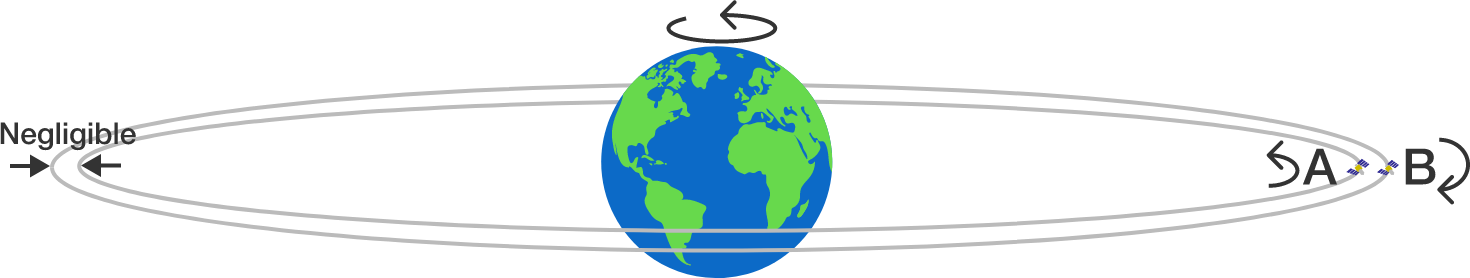# Satellites in orbitTwo identical satellites $A$ and $B$ are launched in equatorial circular orbits around Earth with the same period of revolution of 4.8 hours. Satellite $A$ rotates west to east (with Earth's rotation) while satellite $B$ rotates east to west (against Earth's rotation).

On a particular day, both satellites were seen directly overhead by an observer standing at point $P$ on Earth’s equator. How long (in hours) will it be until both satellites are seen directly overhead again from the same point $P?$


Details and Assumptions:

• The orbits are located on nearly the same circle, with just enough separation to avoid collision.
×